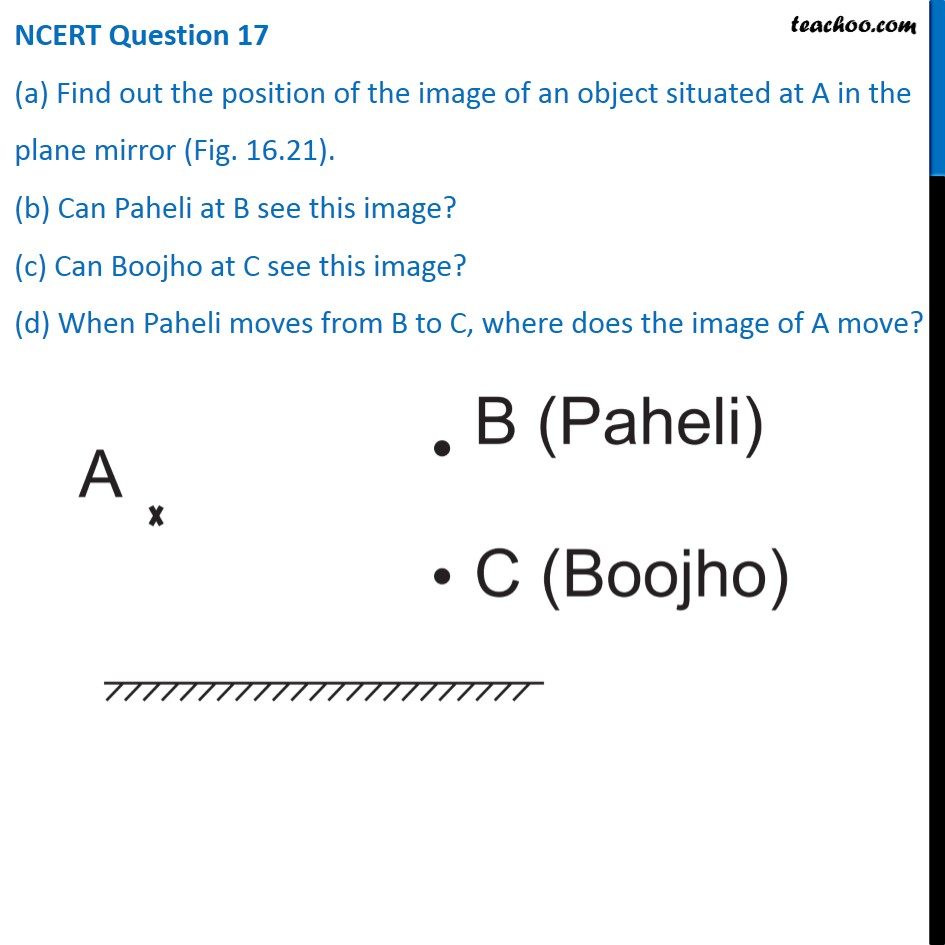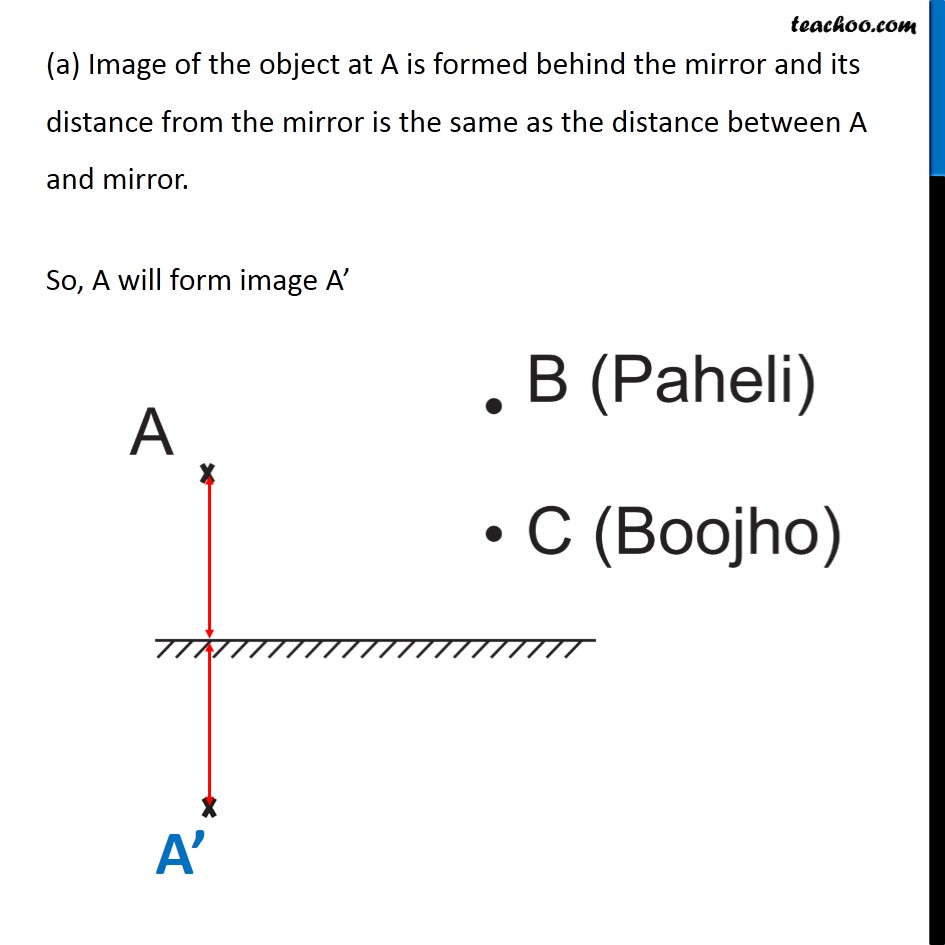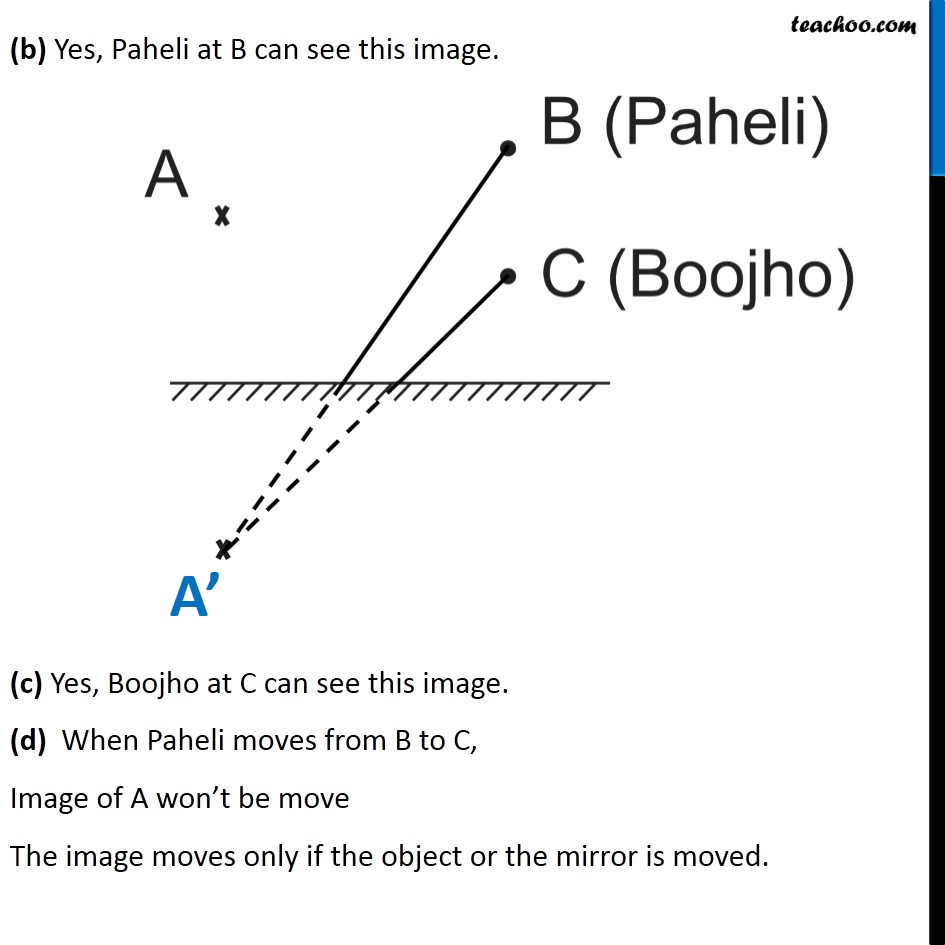1. Class 8
2. Chapter 16 Class 8 - Light
3. NCERT Questions

Transcript

NCERT Question 17 (a) Find out the position of the image of an object situated at A in the plane mirror (Fig. 16.21). (b) Can Paheli at B see this image? (c) Can Boojho at C see this image? (d) When Paheli moves from B to C, where does the image of A move? (a) Image of the object at A is formed behind the mirror and its distance from the mirror is the same as the distance between A and mirror. So, A will form image A’ (b) Yes, Paheli at B can see this image. (c) Yes, Boojho at C can see this image. (d) When Paheli moves from B to C, Image of A won’t be move The image moves only if the object or the mirror is moved.

NCERT Questions

Class 8
Chapter 16 Class 8 - Light